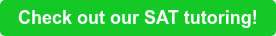# Tackling unfamiliar problems on the SAT math sectionsLet's face it: there's no way to control exactly what math questions will pop up on test day. Questions at the beginning of each section tend to be simple and straightforward--you might be asked to isolate a variable, determine the slope between two points, or solve a system of equations--but later questions can often feel like they've come out of left field, even if you've spent hours completing practice sections.

It's important to become a flexible, creative problem-solver if you're looking to master the trickiest questions on the SAT math sections. Throughout test prep, you should aim to build a "math toolbox" from which you can pull relevant formulas and factoids--anything from the quadratic formula to SOH CAH TOA. And once you've got your toolbox assembled, it is essential that you're able to identify what tools a problem might require.

Categorizing problems is a skill that requires practice. Try asking yourself, why did the makers of the SAT include this particular problem on the test? You could also think of it as "hashtagging" the problems, like you'd hashtag an Instagram post.

There are four easy steps to remember when categorizing an SAT math question:

## 1.Find context clues

Every problem will have key words and symbols that will reveal the category of problem. Your job is to look at every problem as a detective would – searching for clues first!

## 2.Interpret the clues

In order to be able to categorize the problem type, you’ll need to interpret the clues! What kinds of problems are affiliated with the keys words and symbols? What do they make you think of?

## 3.Categorize!

Once you’ve interpreted the clues you identified, you should categorize your problem. Use the hashtag symbol followed by the problem type.

## 4.Solve it.

Now that you’ve identified the problem type, you can carefully choose the math tools in your toolbox to use when solving the problem!

Let's take a look at a couple of problems to see how we might categorize them:

(SAT practice problems included are from an official, released college board PSAT)

## Problem 11. Find the context clues.

I can see that there are rates (per hour) and fixed fees mentioned for each of the gardeners. The phrase “greater than” is also used, and the answer choices are written as inequalities.

2. Interpret the context clues

Interpret the context clues by using the phrase “___ makes me think of ___.” For example, rate makes me think of slope, and fixed fees make me think of y-intercepts.

3. Categorize!

Since slope and y-intercepts are involved, this must be a linear model problem. There are also two different models, which means that this problem must involve a system of equations. Plus, I’ve already identified the presence of inequalities. #linearmodel #systemsofequations #inequalities

4. Solve it.

Now that I’ve categorized the problem, I know to solve a system of linear inequalities using whichever method I’m most comfortable with.

## Problem 21. Find the context clues

I am given an isosceles triangle with congruent legs AB and AC, and I’m also given a ratio.

2. Interpret the context clues

The word ratio in a triangle problem makes me think of similar triangles. I also know that the larger isosceles triangle must have congruent base angles.

3. Categorize!

#similartriangles #sideratios #AAAcongruence

4. Solve it.

Now I know that I can draw the two smaller triangles side by side, match up their congruent angles, label the side lengths in terms of x, and solve as I would any other similar triangle problem.

While you will need to be comfortable with your math content to finish the test, this process of categorizing can help to get you started with any problems that may look unfamiliar.

Feeling unsure about fundamental key words or symbols on the math section of the SAT? You should contact Cambridge Coaching. Our tutors can help you strengthen fundamental concepts so you can move quickly through problems on this section. We’ll build a customized syllabus that maps on to your strengths and weaknesses!